login  home  contents  what's new  discussion  bug reports help  links  subscribe  changes  refresh  edit

(the maths inside is not meant to be taken seriously; 'tis a silly idea that can't work)

Note that dropping comutativity below we get quantum probablility. Franz Lehner added packages supporting computations for several popular versions. In principle one can treat commutative case as a special case of quantum probablility...

from a recent email by Peter Broadbery

Random variables are assumed to have the following properties:

1. complex constants are random variables;
2. the sum of two random variables is a random variable;
3. the product of two random variables is a random variable;
4. addition and multiplication of random variables are both commutative; and
5. there is a notion of conjugation of random variables, satisfying: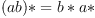and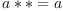for all random variables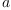,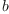, and coinciding with complex conjugation ifis a constant.

This means that random variables form complex abelian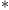-algebras. If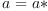, the random variable a is called "real".

An expectation E on an algebra A of random variables is a normalized, positive linear functional. What this means is that

1.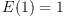;
2.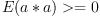for all random variables;
3.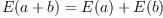for all random variablesand; and
4.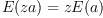if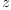is a constant.

##-algebra

In mathematics, a-algebra is an associative algebra over the field of complex numbers with an antilinear, antiautomorphism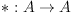which is an involution. More precisely,is required to satisfy the following properties:

•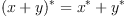•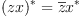•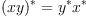•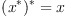for all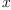,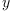in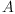, and allin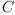.

The most obvious example of a-algebra is the field of complex numbers C whereis just complex conjugation. Another example is the algebra of nn matrices overwithgiven by the conjugate transpose.

An algebra homomorphism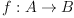is a-homomorphism if it is compatible with the involutions ofand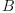, i.e.

•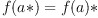for allin.

An elementinis called self-adjoint if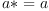.

fricas
(1) -> <aldor>
#include "fricas"
RandomAlgebra(F: Field): Category == with {
Algebra F;
E: % -> F;
sample: % -> F;
}
local PolyHelper(F: Field): with {
expand: SparseUnivariatePolynomial F -> Generator Cross(F, NonNegativeInteger);
}
expand(p: SparseUnivariatePolynomial F): Generator Cross(F, NonNegativeInteger) == generate {
default m: SparseUnivariatePolynomial F;
import from SparseUnivariatePolynomial F;
import from List SparseUnivariatePolynomial F;
for m in monomials p repeat {
}
}
}
UnivariateNormalRandomAlgebra: RandomAlgebra Float with {
X: () -> %;
variance: % -> Float;
Rep ==> SparseUnivariatePolynomial Float;
import from Rep;
0: % == per 0;
1: % == per 1;
X(): % == per(monomial(1$Float,1$NonNegativeInteger)$Rep); characteristic(): NonNegativeInteger == 0; -(x: %): % == per(-rep x); (a: %) = (b: %): Boolean == rep(a) = rep(b); (a: %) + (b: %): % == per(rep(a) + rep(b)); (a: %) * (b: %): % == per(rep(a) * rep(b)); (a: Float) * (b: %): % == per(a * rep(b)); coerce(x: Integer): % == per(x::Rep); coerce(x: Float): % == per(x::Rep); coerce(x: %): OutputForm == coerce rep(x); E(X: %): Float == { import from PolyHelper Float; z: Float := 0; for p in expand rep(X) repeat { (a, b) := p; z := z + a * E(b); } z } -- should be a random sampling of x. sample(X: %): Float == { import from PolyHelper Float; import from Float; u := uniform01()$RandomFloatDistributions;
x: Float := 0;
for p in expand rep(X) repeat {
(a, b) := p;
x := x + a * u^b;
}
return x;
}
variance(X: %): Float == { A := (X-E(X)*1); E(A*A); }
-- return expected value of X^n
local E(n: NonNegativeInteger): Float == {
p: Rep := 1;
-- yuck.  There must be a nicer way than this..
for i in 1..n repeat p := differentiate(p) + monomial(1,1)*p;
coefficient(p,0);
}
}</aldor>
fricas
Compiling FriCAS source code from file
/var/lib/zope2.10/instance/axiom-wiki/var/LatexWiki/5264249576821309342-25px001.as
using Aldor compiler and options
-O -Fasy -Fao -Flsp -lfricas -Mno-ALDOR_W_WillObsolete -DFriCAS -Y $FRICAS/algebra -I$FRICAS/algebra
Use the system command )set compiler args to change these
options.
fricas
Compiling Lisp source code from file
./5264249576821309342-25px001.lsp
Issuing )library command for 5264249576821309342-25px001
fricas
Reading /var/aw/var/LatexWiki/5264249576821309342-25px001.asy
RandomAlgebra is now explicitly exposed in frame initial
RandomAlgebra will be automatically loaded when needed from
/var/aw/var/LatexWiki/5264249576821309342-25px001
UnivariateNormalRandomAlgebra is now explicitly exposed in frame
initial
UnivariateNormalRandomAlgebra will be automatically loaded when
needed from /var/aw/var/LatexWiki/5264249576821309342-25px001

fricas
a := X()\$UnivariateNormalRandomAlgebra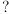(1)
Type: UnivariateNormalRandomAlgebra?
fricas
-- a number, normally distributed
sample a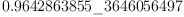(2)
Type: Float
fricas
-- 0
E a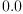(3)
Type: Float
fricas
-- 1
variance(a)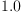(4)
Type: Float
fricas
-- 1
variance(a+5)(5)
Type: Float
fricas
-- 5
variance(a+5)(6)
Type: Float
fricas
-- 3, apparently
variance(a^2 + a)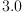(7)
Type: Float

 Subject:   Be Bold !! ( 14 subscribers )